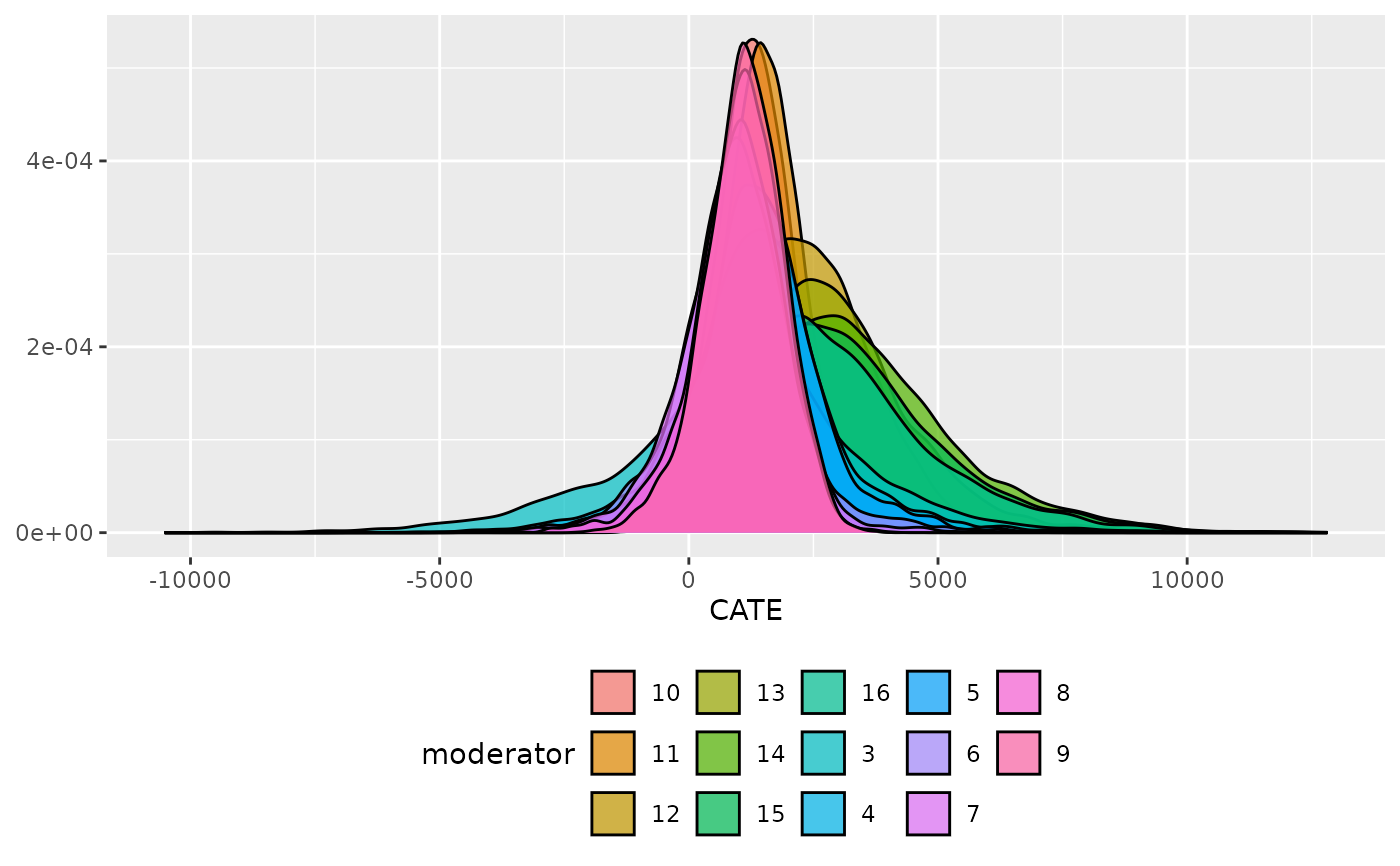Plot the Conditional Average Treatment Effect split by a discrete moderating variable. This plot will provide a visual test of moderation by discrete variables.

## Usage

plot_moderator_d(
.model,
moderator,
type = c("density", "histogram", "errorbar"),
.alpha = 0.7,
facet = FALSE,
.ncol = 1
)

## Arguments

.model

a model produced by bartCause::bartc()

moderator

the moderator as a vector

type

string to specify if you would like to plot a histogram, density or error bar plot

.alpha

transparency value [0, 1]

facet

TRUE/FALSE. Create panel plots of each moderator level?

.ncol

number of columns to use when faceting

ggplot object

George Perrett

## Examples

# \donttest{
data(lalonde)
confounders <- c('age', 'educ', 'black', 'hisp', 'married', 'nodegr')
model_results <- bartCause::bartc(
response = lalonde[['re78']],
treatment = lalonde[['treat']],
confounders = as.matrix(lalonde[, confounders]),
estimand = 'ate',
commonSuprule = 'none'
)
#> fitting treatment model via method 'bart'
#> fitting response model via method 'bart'
plot_moderator_d(model_results, lalonde\$educ)# }